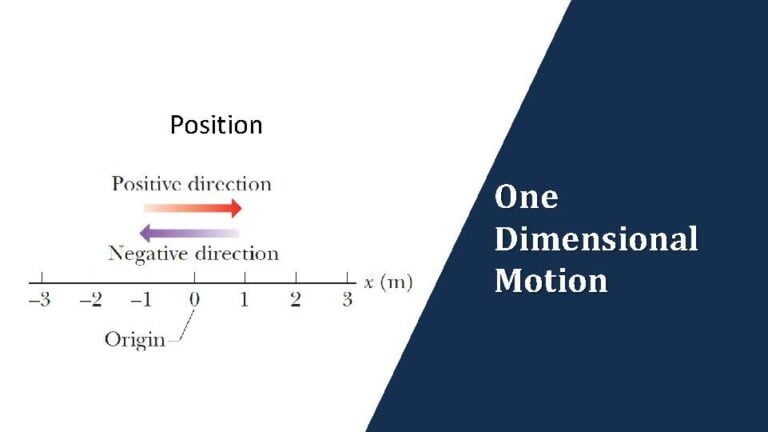Kinematics Questions | Week 1 | One Dimensional MotionWeek 1 – One Dimensional Motion (June 2 – June 8, 2021)

Kinematics questions on one dimensional motion with particle either under a) variable acceleration & b) constant acceleration

Lately in JEE Mains (2020 & 2021), there have been as many questions from 1 dimensional motion as from 2 dimensional motion. They are typically of medium difficulty and you will be able to crack them if you have listened through the videos on question types and solved practice problems for each of those question types.

In JEE Advanced, we have not seen questions on 1-dimensional motion in the last 5 years, but then never underestimate the ability of IIT faculty in coming up with questions on seemingly simpler topics that will test the concepts in creative ways.

So let’s begin with an overview of what to expect in week 1

Kinematics | Questions Types on Variable Acceleration

Here are the five different types of kinematics questions you can expect on variable acceleration in JEE Mains and JEE Advanced. Take a note of question type wherein acceleration is a function of position x i.e. a = f(x)

Listen through the lecture videos wherein Vikas Sir will walk you through the steps to solve such questions and then discuss a sample problem from the latest JEE Main and JEE Advanced exam papers

Question type 1:

Variable $a$ | Given either position $x$, or velocity, $v$ or acceleration, $a$, as a function of time $t$, determine the other kinematic variable(s)

Sample practice problem on this question type: (For more practice problems on this question type, please sign-in)

The distance $x$ covered by a particle in one dimension motion varies with time $t$ as $x^2 = at^2 + 2bt + c$, where $a$, $b$, $c$ are constants. Acceleration of particle depend on $x$ as $x^{-n}$, the value of $n$ is…

Remaining question types on variable acceleration that you should complete this week: (Click here to sign-in and access these question types)

2. Given speed, v, vs time, t, graph, determine the unknown

3. Given acceleration, a, as a function of time, t, determine change in velocity over a given time duration

4. Given acceleration, a, as a function of position, x, determine the velocity of the particle at given position $x$

5. Given acceleration, a, as a function of velocity, v determine the time duration for given initial and final velocities

Kinematics | Question types on constant acceleration

Here are the four different types of kinematics questions you can expect on constant acceleration in JEE Mains and JEE Advanced. Especially watch out for the question type wherein particle’s initial velocity is opposite to the direction of its acceleration which means if the particle reverses direction during the period of interest, then distance will not be the same or equal to the displacement

Question type 6:

1D Motion with constant acceleration – simpler application

A block of mass $m$ is gently placed over a massive plank moving horizontally over a smooth surface with velocity $10$ $m/s$. The coefficient of friction between the block and the plank is $0.2$. The distance travelled by the block till it slides on the plank is [$g = 10 \ m/s^2$]

(a) $10 \ m$  (b) $15 \ m$  (c) $25 \ m$  (d) $35 \ m$

Remaining question types on constant acceleration that you should complete this week: (Click here to sign-in and access these question types)

7. 1D Motion in same direction – 2 or more sections with same a

8. 1D Motion with change in direction – 2 or more segments with same a

9. 1D Motion in same direction – 2 segments with different a